Do you like the article?
Share it with others -
post a link to it!
Use new possibilities of MetaTrader 5

# The 'Turtle Soup' trading system and its 'Turtle Soup Plus One' modification

5 December 2016, 15:30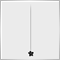2
24 236

### Introduction

The authors of Street Smarts: High Probability Short-Term Trading Strategies Laurence Connors and Linda Raschke are successful traders with the total of 34 years of trading experience. Their extensive experience includes trading on stock exchanges, as well as related positions in banks, hedge funds, brokerage companies and consulting firms. They believe that you only need one trading strategy (TS) for stable and profitable trading. However, the book contains nearly two dozen of TS variants, divided into four groups. Each group refers to a specific phase of market cycles, and operates with one of the stable price behavior patterns.

The strategies described in the book are quite popular. But it is important to understand that the authors have developed them based on the 15...20 year old market behavior. So, the article has two goals — we will implement in MQL5 the first trading strategy described in the book, and then we will try to evaluate its efficiency using the MetaTrader 5 Strategy Tester. We will use the price history of recent years available on MetaQuotes' demo server.

When writing the code, I will address MQL5 users with a basic knowledge of the language, i.e. slightly advanced beginners. Therefore, the article does not contain explanation of how standard functions work, why these types of variables are used, and of all other details that users usually study and practice before starting to program Expert Advisors. On the other hand, I will not address experienced trading robot developers, since they already have well tested libraries of their own solutions, which they use when implementing new trading strategies.

Most of programmers, to whom the article is intended, are interested in studying object-oriented programming. So I will try to make the development of this Expert Adviser useful for the above mentioned purpose. In order to make the transition from the procedural to the object-oriented approach easier, we do not use the most complicated part of OOP — classes. Instead, we will use their simple analogue — structures. Structures join logically connected data of different types and functions for working with them. They possess almost all features of a class including inheritance. But you can use them without knowing the rules of class code formatting, you can do a minimum of adjustments to what you usually do in the procedural programming.

### The 'Turtle Soup' trading system and its 'Turtle Soup Plus One' modification

Turtle Soup is the first trading strategy in the series called 'Tests'. In order to make it clearer, on what basis the series is chosen, the series should have been called 'Testing range limits or support/resistance levels using the price'. Turtle Soup is based on the assumption that the price cannot break a 20-day range without a bounce from the borders of the range. Our task is to try to profit from a temporary bounce or a false breakout. A trading position will always be directed inside the channel, so the trading strategy can be called a "bounce strategy".

By the way, the similarity of the name Turtle Soup and the famous Turtles strategy is not accidental — both strategies monitor price action at the limits of a 20-day range. The book authors have tried to use a couple of breakout strategies, including "Turtles", but such trading was inefficient due to a large number of false signals and deep rollbacks. But they revealed some patterns that helped to create a set of rules to profit from price movements in the direction opposite to the breakout.

A complete set of Buy trade entry rules in the "Turtle Soup" TS can be formulated as follows:

1. Make sure that at least three days have passed since the previous 20-day low
2. Wait until the instrument price falls below the 20-day low
3. Place a pending buy order 5-10 points above the recently broken down price low
4. Once the pending order triggers, set its StopLoss at 1 point below this day's low price
5. Use a Trailing Stop once the position becomes profitable
6. If the position closes by a Stop Loss on the first or second day, you can repeat the entry at the initial level

Sell trade rules are similar, they should be applied at the upper border of the range, i.e. based on the 20-day high.

One of the indicators available in the Code Base can display channel borders on each history bar with appropriate settings. You can use this indicator for visualization in manual trading.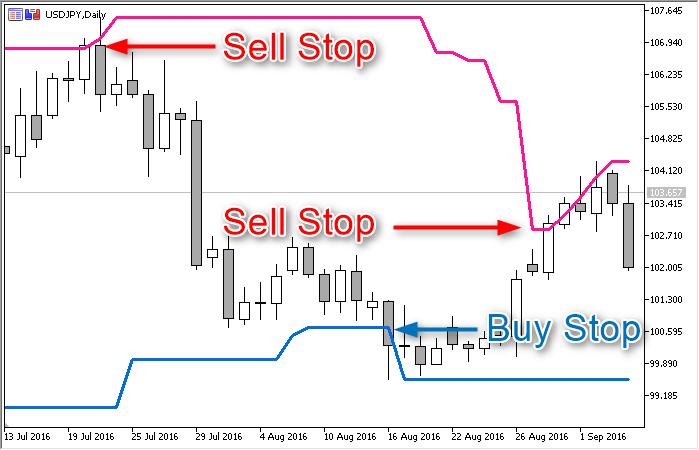The TS description does not provide a direct answer to the question about how long you should keep the pending order, so let's use a simple logic. When testing the range border, the price will create a new extreme point, and the first of the above conditions will become impossible the next day. Since there will be no signal on that day, we will have to cancel the pending order of the previous day.

A modified version of this TS called 'Turtle Soup Plus One' has two differences:

1. Instead of placing a pending order immediately after the breakout of the 20-day range, you should wait for a confirmation signal — this day's bar should close out of the range. The day closure on the border of the analyzed horizontal channel is also ok.
2. To determine the level of the initial StopLoss, we use the appropriate two-day extreme (high or low) price.

### Defining Channel Parameters

To check the conditions, we need to know the high and low price of the range, which can be found after defining the time limits. These four variables determine the channel at any given time, so they can be combined into a single structure. Let's add to it two more variables used in the TS, including the number of days (bars) passed since the low and high of the range:

```struct CHANNEL {
double    d_High;           // The price of the upper range border
double    d_Low;            // The price of the lower range border
datetime  t_From;           // The date/time of the first (oldest) bar of the channel
datetime  t_To;             // The date/time of the last bar of the channel
int       i_Highest_Offset; // The number of bars to the right of the High
int       i_Lowest_Offset;  // The number of bars to the right of the Low
};

```

All these variables will be promptly updated by the f_Set function. The function needs to know on which bar it should start to draw a virtual channel (i_Newest_Bar_Shift) and the depth of the history it should view (i_Bars_Limit):

```void f_Set(int i_Bars_Limit, int i_Newest_Bar_Shift = 1) {
double da_Price_Array[]; // An auxiliary array for the High/Low prices of all bars of the channel

// Determining the upper border of the channel:

int i_Price_Bars = CopyHigh(_Symbol, PERIOD_CURRENT, i_Newest_Bar_Shift, i_Bars_Limit, da_Price_Array);
int i_Bar = ArrayMaximum(da_Price_Array);
d_High = da_Price_Array[i_Bar]; // The upper channel of the range is determined
i_Highest_Offset = i_Price_Bars - i_Bar; // The age of the High (in bars)

// Determining the lower border of the range:

i_Price_Bars = CopyLow(_Symbol, PERIOD_CURRENT, i_Newest_Bar_Shift, i_Bars_Limit, da_Price_Array);
i_Bar = ArrayMinimum(da_Price_Array);
d_Low = da_Price_Array[i_Bar]; // The lower channel of the range is determined
i_Lowest_Offset = i_Price_Bars - i_Bar; // The age of the Low (in bars)

datetime ta_Time_Array[];
i_Price_Bars = CopyTime(_Symbol, PERIOD_CURRENT, i_Newest_Bar_Shift, i_Bars_Limit, ta_Time_Array);
t_From = ta_Time_Array;
t_To = ta_Time_Array[i_Price_Bars - 1];
}

```

This function code has only 13 lines; but if you have read in the language Reference the explanation of MQL functions that access data of timeseries (CopyHigh, CopyLow, CopyTime and others), you know that they are not so simple. In some cases, the number of values returned by the function may differ from what you request, because the requested data may not be ready when you first access the desired timeseries. Data copying from timeseries can work the way you want it with a proper handling of results.

Therefore, let's follow at least the minimum criteria for quality programming and let's add simple error handlers. To make errors easier to understand, let's print error data to log. Logging is also very useful for debugging, because it allows having detailed information about why the order has taken a certain decision. Let us introduce a new variable of an enumeration type, which will set how many details our log should contain:

```enum ENUM_LOG_LEVEL { // The list of logging levels
LOG_LEVEL_NONE,     // Logging disabled
LOG_LEVEL_ERR,      // Only error information
LOG_LEVEL_INFO,     // Errors + robot's comments
LOG_LEVEL_DEBUG     // Everything
};
```

The required level will be selected by the user, and the appropriate operators that print information to log will be added to many functions. Therefore both the list and the custom variable Log_Level should be included into the beginning of the main program rather than the signal block.

Let's get back to the f_Set function, which will look like this with all additional checks (the added lines are highlighted):

```void f_Set(int i_Bars_Limit, int i_Newest_Bar_Shift = 1) {
double da_Price_Array[]; // An auxiliary array for the High/Low prices of all bars of the channel

// Determining the upper border of the channel:

int i_Price_Bars = CopyHigh(_Symbol, PERIOD_CURRENT, i_Newest_Bar_Shift, i_Bars_Limit, da_Price_Array);

if(i_Price_Bars == WRONG_VALUE) {
// Handling the CopyHigh function error
if(Log_Level > LOG_LEVEL_NONE) PrintFormat("%s: CopyHigh: error #%u", __FUNCSIG__, _LastError);
return;
}

if(i_Price_Bars < i_Bars_Limit) {
// The CopyHigh function has not retrieved the required data amount
if(Log_Level > LOG_LEVEL_NONE) PrintFormat("%s: CopyHigh: copied %u bars of %u", __FUNCSIG__, i_Price_Bars, i_Bars_Limit);
return;
}

int i_Bar = ArrayMaximum(da_Price_Array);
if(i_Bar == WRONG_VALUE) {
// Handling the ArrayMaximum function error
if(Log_Level > LOG_LEVEL_NONE) PrintFormat("%s: ArrayMaximum: error #%u", __FUNCSIG__, _LastError);
return;
}

d_High = da_Price_Array[i_Bar]; // The upper channel of the range is determined
i_Highest_Offset = i_Price_Bars - i_Bar; // The age of the High (in bars)

// Determining the lower border of the range:

i_Price_Bars = CopyLow(_Symbol, PERIOD_CURRENT, i_Newest_Bar_Shift, i_Bars_Limit, da_Price_Array);

if(i_Price_Bars == WRONG_VALUE) {
// Handling the CopyLow function error
if(Log_Level > LOG_LEVEL_NONE) PrintFormat("%s: CopyLow: error #%u", __FUNCSIG__, _LastError);
return;
}

if(i_Price_Bars < i_Bars_Limit) {
// The CopyLow function has not retrieved the required data amount
if(Log_Level > LOG_LEVEL_NONE) PrintFormat("%s: CopyLow: copied %u bars of %u", __FUNCSIG__, i_Price_Bars, i_Bars_Limit);
return;
}

i_Bar = ArrayMinimum(da_Price_Array);
if(i_Bar == WRONG_VALUE) {
// Handling the ArrayMinimum function error
if(Log_Level > LOG_LEVEL_NONE) PrintFormat("%s: ArrayMinimum: error #%u", __FUNCSIG__, _LastError);
return;
}
d_Low = da_Price_Array[i_Bar]; // The lower channel of the range is determined
i_Lowest_Offset = i_Price_Bars - i_Bar; // The age of the Low (in bars)

datetime ta_Time_Array[];
i_Price_Bars = CopyTime(_Symbol, PERIOD_CURRENT, i_Newest_Bar_Shift, i_Bars_Limit, ta_Time_Array);
if(i_Price_Bars < 1) t_From = t_To = 0;
else {
t_From = ta_Time_Array;
t_To = ta_Time_Array[i_Price_Bars - 1];
}
}
```

When an error is detected, we do the following: break execution so that the terminal can download the required data for the copy function till the next tick. In order to prevent other functions from using the channel until the procedure fully completes, let us add to the structure the appropriate flag b_Ready (true = data are ready, false = the process has not completed yet). We will also add the channel parameters update flag (b_Updated) — for the optimal performance, it is useful to know if the four parameters used in the TS have change. For this purpose we need to add one more variable — the channel signature (s_Signature). The f_Set function should also be added to the structure, and the CHANNEL structure will look like this:

```// Channel information and functions for collecting and updating it, in one structure
struct CHANNEL {
// Variables
double    d_High;           // The price of the upper range border
double    d_Low;            // The price of the lower range border
datetime  t_From;           // The date/time of the first (oldest) bar of the channel
datetime  t_To;             // The date/time of the last bar of the channel
int       i_Highest_Offset; // The number of bars to the right of the High
int       i_Lowest_Offset;  // The number of bars to the right of the Low
bool      b_Ready;          // Is the parameters update procedure over?
bool      b_Updated;        // Have the channel parameters changed?
string    s_Signature;      // The signature of the last known set of data

// Functions:

CHANNEL() {
d_High = d_Low = 0;
t_From = t_To = 0;
b_Ready = b_Updated = false;
s_Signature = "-";
i_Highest_Offset = i_Lowest_Offset = WRONG_VALUE;
}

void f_Set(int i_Bars_Limit, int i_Newest_Bar_Shift = 1) {
b_Ready = false; // Pitstop: set a service flag

double da_Price_Array[]; // An auxiliary array for the High/Low prices of all bars of the channel

// Determining the upper border of the channel:

int i_Price_Bars = CopyHigh(_Symbol, PERIOD_CURRENT, i_Newest_Bar_Shift, i_Bars_Limit, da_Price_Array);
if(i_Price_Bars == WRONG_VALUE) {
// Handling the CopyHigh function error
if(Log_Level > LOG_LEVEL_NONE) PrintFormat("%s: CopyHigh: error #%u", __FUNCSIG__, _LastError);
return;
}

if(i_Price_Bars < i_Bars_Limit) {
// The CopyHigh function has not retrieved the required data amount
if(Log_Level > LOG_LEVEL_NONE) PrintFormat("%s: CopyHigh: copied %u bars of %u", __FUNCSIG__, i_Price_Bars, i_Bars_Limit);
return;
}

int i_Bar = ArrayMaximum(da_Price_Array);
if(i_Bar == WRONG_VALUE) {
// Handling the ArrayMaximum function error
if(Log_Level > LOG_LEVEL_NONE) PrintFormat("%s: ArrayMaximum: error #%u", __FUNCSIG__, _LastError);
return;
}

d_High = da_Price_Array[i_Bar]; // The upper channel of the range is determined
i_Highest_Offset = i_Price_Bars - i_Bar; // The age of the High (in bars)

// Determining the lower border of the range:

i_Price_Bars = CopyLow(_Symbol, PERIOD_CURRENT, i_Newest_Bar_Shift, i_Bars_Limit, da_Price_Array);

if(i_Price_Bars == WRONG_VALUE) {
// Handling the CopyLow function error
if(Log_Level > LOG_LEVEL_NONE) PrintFormat("%s: CopyLow: error #%u", __FUNCSIG__, _LastError);
return;
}

if(i_Price_Bars < i_Bars_Limit) {
// The CopyLow function has not retrieved the required data amount
if(Log_Level > LOG_LEVEL_NONE) PrintFormat("%s: CopyLow: copied %u bars of %u", __FUNCSIG__, i_Price_Bars, i_Bars_Limit);
return;
}

i_Bar = ArrayMinimum(da_Price_Array);
if(i_Bar == WRONG_VALUE) {
// Handling the ArrayMinimum function error
if(Log_Level > LOG_LEVEL_NONE) PrintFormat("%s: ArrayMinimum: error #%u", __FUNCSIG__, _LastError);
return;
}
d_Low = da_Price_Array[i_Bar]; // The lower channel of the range is determined
i_Lowest_Offset = i_Price_Bars - i_Bar; // The age of the Low (in bars)

datetime ta_Time_Array[];
i_Price_Bars = CopyTime(_Symbol, PERIOD_CURRENT, i_Newest_Bar_Shift, i_Bars_Limit, ta_Time_Array);
if(i_Price_Bars < 1) t_From = t_To = 0;
else {
t_From = ta_Time_Array;
t_To = ta_Time_Array[i_Price_Bars - 1];
}

string s_New_Signature = StringFormat("%.5f%.5f%u%u", d_Low, d_High, t_From, t_To);
if(s_Signature != s_New_Signature) {
// Channel data have changed
b_Updated = true;
if(Log_Level > LOG_LEVEL_ERR) PrintFormat("%s: Channel updated: %s .. %s / %s .. %s, min: %u max: %u ", __FUNCTION__, DoubleToString(d_Low, _Digits), DoubleToString(d_High, _Digits), TimeToString(t_From, TIME_DATE|TIME_MINUTES), TimeToString(t_To, TIME_DATE|TIME_MINUTES), i_Lowest_Offset, i_Highest_Offset);
s_Signature = s_New_Signature;
}

b_Ready = true; // Data update successfully completed
}
};
```
Now we need to declare one channel object of this type on the global level (to make it accessible from various user functions):

`CHANNEL go_Channel;`

### Signal Generation Function

According to this system, a buy signal is determined by two required conditions:

1. At least three trading days have passed since the last 20-day low

2a. The symbol price has fallen below the 20-day low (Turtle Soup)

2b. The daily bar has closed not higher than the 20-day low (Turtle Soup Plus One)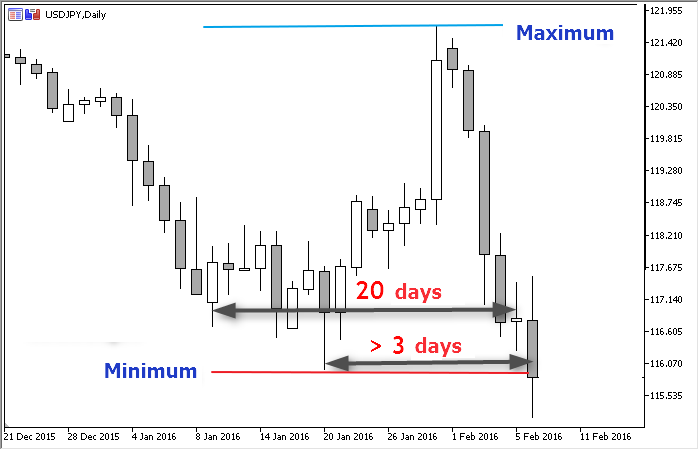All other TS rules described above belong to the trade order parameters and position management, so we will not include them into the signal block.

In one module we will program signals according to the rules of both modifications of the trading system (Turtle Soup and Turtle Soup Plus One). The possibility to select the appropriate version of the rules will be added to the Expert Advisor parameters. Let's call the appropriate custom variable Strategy_Type. In our case the list of strategies contains only to options, so using true/false (a bool type variable) would be easier. But we will need the possibility to add all strategies translated to code within this series of articles, so let's use a numbered list:

```enum ENUM_STRATEGY {     // The list of strategies
TS_TURTLE_SOUP,        // Turtle Soup
TS_TURTLE_SOUP_PLUS_1  // Turtle Soup Plus One
};
input ENUM_STRATEGY  Strategy_Type = TS_TURTLE_SOUP;  // Trading Strategy:
```

The type of the strategy should be passed to the signal detection functions of the main program, i.e. it needs to know whether to wait for a bar (day) close — the b_Wait_For_Bar_Close variable of the bool type. The second required variable is the pause after the previous extremum i_Extremum_Bars. The function should return the signal status: whether buy/sell conditions are met or it should wait. An appropriate numbered list will also be added to the main Expert Advisor file:

```enum ENUM_ENTRY_SIGNAL {  // The list of entry signals
ENTRY_BUY,              // A Buy signal
ENTRY_SELL,             // A Sell signal
ENTRY_NONE,             // No signal
ENTRY_UNKNOWN           // An indefinite status
};
```

Another structure that both the signal module and functions of the main program will use is the go_Tick global object containing information about the most recent tick. This is a standard structure of the MqlTick type, which should be declared in the main file. Later we will program its update in the main program body (the OnTick function).

`MqlTick go_Tick; // Information about the last known tick`

Now, finally, we can proceed to the main function of the module

```ENUM_ENTRY_SIGNAL fe_Get_Entry_Signal(
bool b_Wait_For_Bar_Close = false,
int i_Extremum_Bars = 3
) {}
```

Let's start with the check of a Sell signal condition; whether enough days (bars) have passed after the previous High (the first condition), and whether the price has broken the upper range limit (the second condition):

```if(go_Channel.i_Highest_Offset > i_Extremum_Bars) // 1st condition
if(go_Channel.d_High < d_Actual_Price) // 2nd condition
return(ENTRY_SELL); // Both Sell conditions are met
```

Check of the Buy signal conditions is similar:

```if(go_Channel.i_Lowest_Offset > i_Extremum_Bars) // 1st condition
if(go_Channel.d_Low > d_Actual_Price) { // 2nd condition
return(ENTRY_BUY); // Both Buy conditions are met
```

Here we have used the d_Actual_Price variable that contains the current price relevant for this TS. For Turtle Soup this means the last known bid price, for Turtle Soup Plus One it is the previous day's (bar's) close price:

```double d_Actual_Price = go_Tick.bid; // The default price - for the Turtle Soup version
if(b_Wait_For_Bar_Close) { // for the Turtle Soup Plus One version
double da_Price_Array; // An auxiliary array
CopyClose(_Symbol, PERIOD_CURRENT, 1, 1, da_Price_Array));
d_Actual_Price = da_Price_Array;
}
```

The function that includes the minimum required actions looks like this:

```ENUM_ENTRY_SIGNAL fe_Get_Entry_Signal(bool b_Wait_For_Bar_Close = false, int i_Extremum_Bars = 3) {
double d_Actual_Price = go_Tick.bid; // The default price - for the Turtle Soup version
if(b_Wait_For_Bar_Close) { // for the Turtle Soup Plus One version
double da_Price_Array;
CopyClose(_Symbol, PERIOD_CURRENT, 1, 1, da_Price_Array));
d_Actual_Price = da_Price_Array;
}

// Upper limit:
if(go_Channel.i_Highest_Offset > i_Extremum_Bars) // 1st condition
if(go_Channel.d_High < d_Actual_Price) { // 2nd condition
// The price has broken the upper limit
return(ENTRY_SELL);
}

// lower limit:
if(go_Channel.i_Lowest_Offset > i_Extremum_Bars) // 1st condition
if(go_Channel.d_Low > d_Actual_Price) { // 2nd condition
// The price has broken the lower limit
return(ENTRY_BUY);
}

return(ENTRY_NONE);
}
```

Remember that the channel object may not be prepared for data reading from it (flag go_Channel.b_Ready = false). So, we need to add a check of this flag. In this function, we use one of the standard functions for copying data from a timeseries (CopyClose), so let's add possible error handling. Don't forget about logging of significant data, which facilitates debugging:

```ENUM_ENTRY_SIGNAL fe_Get_Entry_Signal(bool b_Wait_For_Bar_Close = false, int i_Extremum_Bars = 3) {
if(!go_Channel.b_Ready) {
// Channel data are not prepared for use
if(Log_Level == LOG_LEVEL_DEBUG) PrintFormat("%s: Channel parameters are not prepared", __FUNCTION__);
return(ENTRY_UNKNOWN);
}

double d_Actual_Price = go_Tick.bid; // The default price - for the Turtle Soup version
if(b_Wait_For_Bar_Close) { // for the Turtle Soup Plus One version
double da_Price_Array;
if(WRONG_VALUE == CopyClose(_Symbol, PERIOD_CURRENT, 1, 1, da_Price_Array)) {
// Handling the error of the CopyClose function
if(Log_Level > LOG_LEVEL_NONE) PrintFormat("%s: CopyClose: error #%u", __FUNCSIG__, _LastError);
return(ENTRY_NONE);
}
d_Actual_Price = da_Price_Array;
}

// Upper limit:
if(go_Channel.i_Highest_Offset > i_Extremum_Bars) // 1st condition
if(go_Channel.d_High < d_Actual_Price) { // 2nd condition
// The price has broken the upper limit
if(Log_Level == LOG_LEVEL_DEBUG) PrintFormat("%s: Price (%s) has broken the upper limit (%s)", __FUNCTION__, DoubleToString(d_Actual_Price, _Digits), DoubleToString(go_Channel.d_High, _Digits));
return(ENTRY_SELL);
}

// lower limit:
if(go_Channel.i_Lowest_Offset > i_Extremum_Bars) // 1st condition
if(go_Channel.d_Low > d_Actual_Price) { // 2nd condition
// The price has broken the lower limit
if(Log_Level == LOG_LEVEL_DEBUG) PrintFormat("%s: Price (%s) has broken the lower limit (%s)", __FUNCTION__, DoubleToString(d_Actual_Price, _Digits), DoubleToString(go_Channel.d_Low, _Digits));
return(ENTRY_BUY);
}

// If the program has reached this line, then the price is inside the range, i.e. the second condition is not satisfied

return(ENTRY_NONE);
}
```

This function will be called with every tick, i.e. hundreds of thousands of times per day. However, if the first condition (not less than three days from the last extremum) is not satisfied, further actions become meaningless. Following rules of proper programming style, we need to minimize resource consumption, so let our function sleep until the next bar (day), i.e. until the update of the channel parameters:

```ENUM_ENTRY_SIGNAL fe_Get_Entry_Signal(bool b_Wait_For_Bar_Close = false, int i_Extremum_Bars = 3) {
static datetime st_Pause_End = 0; // The time of the next check
if(st_Pause_End > go_Tick.time) return(ENTRY_NONE);
st_Pause_End = 0;

if(go_Channel.b_In_Process) {
// Channel data are not prepared for use
if(Log_Level == LOG_LEVEL_DEBUG) PrintFormat("%s: Channel parameters are not prepared", __FUNCTION__);
return(ENTRY_UNKNOWN);
}
if(go_Channel.i_Lowest_Offset < i_Extremum_Bars && go_Channel.i_Highest_Offset < i_Extremum_Bars) {
// The 1st condition is not met
if(Log_Level == LOG_LEVEL_DEBUG) PrintFormat("%s: the 1st condition is not met", __FUNCTION__);

// Pause until the channel is updated
st_Pause_End = go_Tick.time + PeriodSeconds() - go_Tick.time % PeriodSeconds();

return(ENTRY_NONE);
}

double d_Actual_Price = go_Tick.bid; // The default price - for the Turtle Soup version
if(b_Wait_For_Bar_Close) { // for the Turtle Soup Plus One version
double da_Price_Array;
if(WRONG_VALUE == CopyClose(_Symbol, PERIOD_CURRENT, 1, 1, da_Price_Array)) {
// Handling the error of the CopyClose function
if(Log_Level > LOG_LEVEL_NONE) PrintFormat("%s: CopyClose: error #%u", __FUNCSIG__, _LastError);
return(ENTRY_NONE);
}
d_Actual_Price = da_Price_Array;
}

// Upper limit:
if(go_Channel.i_Highest_Offset > i_Extremum_Bars) // 1st condition
if(go_Channel.d_High < d_Actual_Price) { // 2nd condition
// The price has broken the upper limit
if(Log_Level == LOG_LEVEL_DEBUG) PrintFormat("%s: Price (%s) has broken the upper limit (%s)", __FUNCTION__, DoubleToString(d_Actual_Price, _Digits), DoubleToString(go_Channel.d_High, _Digits));
return(ENTRY_SELL);
}

// lower limit:
if(go_Channel.i_Lowest_Offset > i_Extremum_Bars) // 1st condition
if(go_Channel.d_Low > d_Actual_Price) { // 2nd condition
// The price has broken the lower limit
if(Log_Level == LOG_LEVEL_DEBUG) PrintFormat("%s: Price (%s) has broken the lower limit (%s)", __FUNCTION__, DoubleToString(d_Actual_Price, _Digits), DoubleToString(go_Channel.d_Low, _Digits));
return(ENTRY_BUY);
}

// If the program has reached this line, then the price is inside the range, i.e. the second condition is not satisfied

if(b_Wait_For_Bar_Close) // for the Turtle Soup Plus One version
// Pause until the current bar close
st_Pause_End = go_Tick.time + PeriodSeconds() - go_Tick.time % PeriodSeconds();

return(ENTRY_NONE);
}
```

This is the final code of the function. Let's call the file of the signal module Signal_Turtle_Soup.mqh, add to it the code related to the channel and signals; at the file beginning we add entry fields for the custom settings of the strategy:

```enum ENUM_STRATEGY {     // Strategy version
TS_TURTLE_SOUP,        // Turtle Soup
TS_TURTLE_SOUP_PLIS_1  // Turtle Soup Plus One
};

// Custom settings
input ENUM_STRATEGY  Turtle_Soup_Type = TS_TURTLE_SOUP;  // Turtle Soup: Strategy version
input uint           Turtle_Soup_Period_Length = 20;     // Turtle Soup: Extremum search depth (in bars)
input uint           Turtle_Soup_Extremum_Offset = 3;    // Turtle Soup: A pause after the last extremum (in bars)
input double         Turtle_Soup_Entry_Offset = 10;      // Turtle Soup: Entry: Offset from the extreme level (in points)
input double         Turtle_Soup_Exit_Offset = 1;        // Turtle Soup: Exit: Offset from an opposite extremum (in points)
```

Save this file to the terminal data folder; signal libraries should be stored in MQL5\Include\Expert\Signal.

### A Basic Expert Advisor for TS Testing

Near the beginning of the Expert Advisor code, we add custom settings fields, before these fields we add lists of the enum type used in the settings. Let's divide the settings into two groups — "Strategy Settings" and "Position Opening and Management". First group settings will be included from the signal library file during compilation. So far, we have created one such file. In the next articles we will formalize and program other strategies from the book, and it will be possible to replace (or add) signal modules, including required custom settings.

Now we include at the code beginning the MQL5 standard library file for performing trading operations:

```enum ENUM_LOG_LEVEL {  // The list of logging levels
LOG_LEVEL_NONE,      // Logging disabled
LOG_LEVEL_ERR,       // Only error information
LOG_LEVEL_INFO,      // Errors + robot's comments
LOG_LEVEL_DEBUG      // Everything
};
enum ENUM_ENTRY_SIGNAL {  // The list of entry signals
ENTRY_BUY,              // A Buy signal
ENTRY_SELL,             // A Sell signal
ENTRY_NONE,             // No signal
ENTRY_UNKNOWN           // An indefinite status
};

#include <Trade\Trade.mqh> // Class for performing trading operations

input string  _ = "** Strategy settings:";  // .

#include <Expert\Signal\Signal_Turtle_Soup.mqh> // Signal module

input string  __ = "** Position opening and management:"; // .
input double  Trade_Volume = 0.1;                  // Trade volume
input uint    Trail_Trigger = 100;                 // Trailing: Distance to enable trailing (in points)
input uint    Trail_Step = 5;                      // Trailing: SL moving step (in points)
input uint    Trail_Distance = 50;                 // Trailing: Max distance from the price to SL (in points)
input ENUM_LOG_LEVEL  Log_Level = LOG_LEVEL_INFO;  // Logging mode:
```

The authors do not mention any special money management or risk management techniques for this strategy, therefore we will use a fixed lot size for all trades.

Trailing settings should be entered in points. The introduction of five-digit quotes has lead to some confusion with the units used, so here is a note that one point corresponds to the minimum change in the symbol price. This means that for five-digit quotes one point is equal to 0.00001, while for four-digit quotes it is equal to 0.0001. Not to be confused with pips — pips ignore the real accuracy of quotes, the always translate them into four-digit. I.e. if the minimum price change of a symbol (point) is equal to 0.00001, one pip is equal to 10 points; and if the point is equal to 0.0001, the point and pip values are equal.

The trailing stop function uses these settings on every tick, and recalculation of user defined points into real prices of a symbol is performed hundreds of thousands of times a day, although it does not consume much CPU resources. It would be more correct to recalculate the user entered values once during Expert Advisor initialization, and to save them in global variables for future use. The same can be done for the variables that will be used for lot normalization — server limits on the minimum and maximum size, as well as the change step do not change during Expert Advisor operation, so there is no need to read them each time. Here is the declaration of global variables and the initialization function:

```int
gi_Try_To_Trade = 4, // The number of attempts to send a trade order
gi_Connect_Wait = 2000 // A pause between attempts (in milliseconds)
;
double
gd_Stop_Level, // StopLevel from the server settings converted to the symbol price
gd_Lot_Step, gd_Lot_Min, gd_Lot_Max, // Lot value restrictions from the server settings
gd_Entry_Offset, // Entry: Offset from the extremum in symbol prices
gd_Exit_Offset, // Exit: Offset from the extremum in symbol prices
gd_Trail_Trigger, gd_Trail_Step, gd_Trail_Distance // Trailing parameters converted to the symbol price
;
MqlTick go_Tick; // Information about the last known tick

int OnInit() {
// Converting settings from points to symbol prices:
double d_One_Point_Rate = pow(10, _Digits);
gd_Entry_Offset = Turtle_Soup_Entry_Offset / d_One_Point_Rate;
gd_Exit_Offset = Turtle_Soup_Exit_Offset / d_One_Point_Rate;
gd_Trail_Trigger = Trail_Trigger / d_One_Point_Rate;
gd_Trail_Step = Trail_Step / d_One_Point_Rate;
gd_Trail_Distance = Trail_Distance / d_One_Point_Rate;
gd_Stop_Level = SymbolInfoInteger(_Symbol, SYMBOL_TRADE_STOPS_LEVEL) / d_One_Point_Rate;
// Initialization of lot limits:
gd_Lot_Min = SymbolInfoDouble(_Symbol, SYMBOL_VOLUME_MIN);
gd_Lot_Max = SymbolInfoDouble(_Symbol, SYMBOL_VOLUME_MAX);
gd_Lot_Step = SymbolInfoDouble(_Symbol, SYMBOL_VOLUME_STEP);

return(INIT_SUCCEEDED);
}
```

Note that the MQL5 standard library contains a trailing module of the type we need (TrailingFixedPips.mqh), and we could include it into the code similar to the trading operations performing class. But it does not fully comply with the features of this Expert Advisor, so we will write the trailing code and add it to the Expert Advisor body in the form of a separate custom function:

```bool fb_Trailing_Stop(    // Trailing SL of the current symbol position
double d_Trail_Trigger,  // The distance to enable trailing (in symbol prices
double d_Trail_Step,    // SL trailing step (in symbol prices)
double d_Trail_Distance  // min distance from the price to SL (in symbol prices)</s3>
) {
if(!PositionSelect(_Symbol)) return(false); // No position, nothing to trail

// The basic value for the calculation of the new SL level - current price value:
double d_New_SL = PositionGetDouble(POSITION_PRICE_CURRENT);

if(PositionGetInteger(POSITION_TYPE) == POSITION_TYPE_BUY) { // For a long position
if(d_New_SL - PositionGetDouble(POSITION_PRICE_OPEN) < d_Trail_Trigger)
return(false); // The price has not moved far enough to enable trailing

if(d_New_SL - PositionGetDouble(POSITION_SL) < d_Trail_Distance + d_Trail_Step)
return(false); // Price change less than the set SL trailing step

d_New_SL -= d_Trail_Distance; // New SL level
} else if(PositionGetInteger(POSITION_TYPE) == POSITION_TYPE_SELL) { // For a short position
if(PositionGetDouble(POSITION_PRICE_OPEN) - d_New_SL < d_Trail_Trigger)
return(false); // The price has not moved far enough to enable trailing

if(PositionGetDouble(POSITION_SL) > 0.0) if(PositionGetDouble(POSITION_SL) - d_New_SL < d_Trail_Distance + d_Trail_Step)
return(false); // The price has not moved far enough to enable trailing

d_New_SL += d_Trail_Distance; // New SL level
} else return(false);

// Do server settings allow placing the new SL at this distance from the current price?
if(!fb_Is_Acceptable_Distance(d_New_SL, PositionGetDouble(POSITION_PRICE_CURRENT))) return(false);

CTrade Trade;
Trade.LogLevel(LOG_LEVEL_ERRORS);
// Move SL
Trade.PositionModify(_Symbol, d_New_SL, PositionGetDouble(POSITION_TP));

return(true);
}

bool fb_Is_Acceptable_Distance(double d_Level_To_Check, double d_Current_Price) {
return(
fabs(d_Current_Price - d_Level_To_Check)
>
fmax(gd_Stop_Level, go_Tick.ask - go_Tick.bid)
);
}
```

The check whether placing SL at the new distance is allowed is included into a separate function fb_Is_Acceptable_Distance, which can also be used to validate pending order placing level and the Stop Loss level of an open position.

Now we proceed to the main working area in the Expert Advisor code, which is called by a handler function which handles the new tick arrival event - OnTick. According to the rules of the strategy, if there is an open position, the EA should not search for new signals, therefore we begin with an appropriate check. If a position already exists, the robot has two options: either to calculate and set the initial StopLoss level for a new position, or activate the trailing function, which will determine whether the StopLoss should be moved, and will perform the appropriate operation. Calling the trailing function is easy. As for the StopLoss level calculation, we will use the offset from extremum gd_Exit_Offset entered by the user in points and converted to the symbol prices. The extreme price value can be found using the standard MQL5 functions CopyHigh or CopyLow. The calculated levels should then be validated using the fb_Is_Acceptable_Distance function, and also using the current price value from the go_Tick structure. We will separate these calculations and verifications for BuyStop and SellStop orders:

```if(PositionSelect(_Symbol)) { // There is an open position
if(PositionGetDouble(POSITION_SL) == 0.) { // New position
double
d_SL = WRONG_VALUE, // SL level
da_Price_Array[] // An auxiliary array
;

// Calculate the StopLoss level:
if(PositionGetInteger(POSITION_TYPE) == POSITION_TYPE_BUY) { // For a long position
if(WRONG_VALUE == CopyLow(_Symbol, PERIOD_CURRENT, 0, 1 + (Turtle_Soup_Type == TS_TURTLE_SOUP_PLIS_1), da_Price_Array)) {
// Handling the CopyLow function error
if(Log_Level > LOG_LEVEL_NONE) PrintFormat("%s: CopyLow: error #%u", __FUNCTION__, _LastError);
return;
}
d_SL = da_Price_Array[ArrayMinimum(da_Price_Array)] - gd_Exit_Offset;

// Is the distance from the current price enough?
if(!fb_Is_Acceptable_Distance(d_SL, go_Tick.bid)) {
if(Log_Level > LOG_LEVEL_NONE) PrintFormat("Calculated SL level %s is replaced by the minimum allowable %s", DoubleToString(d_SL, _Digits), DoubleToString(go_Tick.bid + fmax(gd_Stop_Level, go_Tick.ask - go_Tick.bid), _Digits));
d_SL = go_Tick.bid - fmax(gd_Stop_Level, go_Tick.ask - go_Tick.bid);
}

} else { // For a short position
if(WRONG_VALUE == CopyHigh(_Symbol, PERIOD_CURRENT, 0, 1 + (Turtle_Soup_Type == TS_TURTLE_SOUP_PLIS_1), da_Price_Array)) {
// Handling the CopyHigh function error
if(Log_Level > LOG_LEVEL_NONE) PrintFormat("%s: CopyHigh: error #%u", __FUNCTION__, _LastError);
return;
}
d_SL = da_Price_Array[ArrayMaximum(da_Price_Array)] + gd_Exit_Offset;

// Is the distance from the current price enough?
if(!fb_Is_Acceptable_Distance(d_SL, go_Tick.ask)) {
if(Log_Level > LOG_LEVEL_NONE) PrintFormat("Calculated SL level %s is replaced by the minimum allowable %s", DoubleToString(d_SL, _Digits), DoubleToString(go_Tick.ask - fmax(gd_Stop_Level, go_Tick.ask - go_Tick.bid), _Digits));
d_SL = go_Tick.ask + fmax(gd_Stop_Level, go_Tick.ask - go_Tick.bid);
}
}

CTrade Trade;
Trade.LogLevel(LOG_LEVEL_ERRORS);
// Set SL
Trade.PositionModify(_Symbol, d_SL, PositionGetDouble(POSITION_TP));
return;
}

// Trailing
fb_Trailing_Stop(gd_Trail_Trigger, gd_Trail_Step, gd_Trail_Distance);
return;
}
```

In addition to the calculated new tick parameters, we also need to update the channel parameters, which are used for signal detection. Calling the appropriate f_Set function of the go_Channel structure only makes sense after the closure of a bar, while these parameters remain unchanged the rest of the time. The trading robot has one more action linked to the new day (bar) beginning, which is the deletion of an irrelevant yesterday's pending order. Let's program these two actions:

```int
i_Order_Ticket = WRONG_VALUE, // The ticket of a pending order
i_Try = gi_Try_To_Trade, // The number of attempts to perform the operation
i_Pending_Type = -10 // The type of the existing pending order
;
static int si_Last_Tick_Bar_Num = 0; // The number of the previous tick's bar (0 = beginning of calculation in MQL)

// Processing events linked to the beginning if a new day (bar):
if(si_Last_Tick_Bar_Num < int(floor(go_Tick.time / PeriodSeconds()))) {
// Hello new day :)
si_Last_Tick_Bar_Num = int(floor(go_Tick.time / PeriodSeconds()));

// Is there an obsolete pending order?
i_Pending_Type = fi_Get_Pending_Type(i_Order_Ticket);
if(i_Pending_Type == ORDER_TYPE_SELL_STOP || i_Pending_Type == ORDER_TYPE_BUY_STOP) {
// Delete the old order:
if(Log_Level > LOG_LEVEL_ERR) Print("Deleting yesterday's pending order");

CTrade o_Trade;
o_Trade.LogLevel(LOG_LEVEL_ERRORS);
while(i_Try-- > 0) { // Attempts to delete
if(o_Trade.OrderDelete(i_Order_Ticket)) { // Attempt successful
i_Try = -10; // The flag of a successful operation
break;
}
// Attempt failed
Sleep(gi_Connect_Wait); // A pause before the next attempt
}

if(i_Try == WRONG_VALUE) { // Deleting a pending order failed
if(Log_Level > LOG_LEVEL_NONE) Print("Pending order deleting error");
return; // Wait till the next tick
}
}

// Updating the channel parameters:
go_Channel.f_Set(Turtle_Soup_Period_Length, 1 + (Turtle_Soup_Type == TS_TURTLE_SOUP_PLIS_1));
}
```

The fi_Get_Pending_Type function used here returns the type of a pending order, and using the received reference to the i_Order_Ticket variable, it adds the ticket number to it. The order type will be used later for comparing the actual signal direction on this tick, while the ticket is used in case you need to delete the order. If there is no pending order, both values will be equal to WRONG_VALUE. The listing of this function is below:

```int fi_Get_Pending_Type( // Detecting the presence of a pending order of the current symbol
int& i_Order_Ticket // A reference to the ticket of the selected pending order
) {
int
i_Order = OrdersTotal(), // The total number of orders
i_Order_Type = WRONG_VALUE // A variable for the order type
;
i_Order_Ticket = WRONG_VALUE; // The default returned ticket value

if(i_Order < 1) return(i_Order_Ticket); // No orders

while(i_Order-- > 0) { // Checking existing orders
i_Order_Ticket = int(OrderGetTicket(i_Order)); // Reading the ticket
if(i_Order_Ticket > 0)
if(StringCompare(OrderGetString(ORDER_SYMBOL), _Symbol, false) == 0) {
i_Order_Type = int(OrderGetInteger(ORDER_TYPE));
// We only need pending orders:
if(i_Order_Type == ORDER_TYPE_BUY_LIMIT || i_Order_Type == ORDER_TYPE_BUY_STOP || i_Order_Type == ORDER_TYPE_SELL_LIMIT || i_Order_Type == ORDER_TYPE_SELL_STOP)
break; // A pending order has been found
}
i_Order_Ticket = WRONG_VALUE; // Not found yet
}

return(i_Order_Type);
}
```

Now everything is ready to determine the status of a signal. If the TS conditions are not satisfied (the signal will have the status of ENTRY_NONE or ENTRY_UNKNOWN), the operation of the main program on this tick can be completed:

```// Get the signal status:
ENUM_ENTRY_SIGNAL e_Signal = fe_Get_Entry_Signal(Turtle_Soup_Type == TS_TURTLE_SOUP_PLIS_1, Turtle_Soup_Extremum_Offset);
if(e_Signal > 1) return; // No signal
```

If there is a signal, compare it with the direction of the existing pending order if it has already been placed:

```// Finding the type of the pending order and its ticket if we haven't done this yet:
if(i_Pending_Type == -10)
i_Pending_Type = fi_Get_Pending_Type(i_Order_Ticket);

// Do we need a new pending order?
if(
(e_Signal == ENTRY_SELL && i_Pending_Type == ORDER_TYPE_SELL_STOP)
||
(e_Signal == ENTRY_BUY && i_Pending_Type == ORDER_TYPE_BUY_STOP)
) return; // There is a pending order in the direction of the signal

// Do we need to delete the pending order?
if(
(e_Signal == ENTRY_SELL && i_Pending_Type == ORDER_TYPE_BUY_STOP)
||
(e_Signal == ENTRY_BUY && i_Pending_Type == ORDER_TYPE_SELL_STOP)
) { // The direction of the pending order does not match the direction of the signal
if(Log_Level > LOG_LEVEL_ERR) Print("The direction of the pending order does not correspond to the direction of the signal");

i_Try = gi_Try_To_Trade;
while(i_Try-- > 0) { // Attempts to delete
if(o_Trade.OrderDelete(i_Order_Ticket)) { // Attempt successful
i_Try = -10; // The flag of a successful operation
break;
}
// Attempt failed
Sleep(gi_Connect_Wait); // A pause before the next attempt
}

if(i_Try == WRONG_VALUE) { // Deleting a pending order failed
if(Log_Level > LOG_LEVEL_NONE) Print("Pending order deleting error");
return; // Wait till the next tick
}
}
```

Now that we know for sure that we need to place a new pending order, let's calculate its parameters. According to the strategy rules, the order should be placed with an offset inward from the channel limits. StopLoss should be placed at the opposite side of the border near the price extremum of today or of two days ago (depending on the selected strategy version). The StopLoss position should only be calculated after the pending order triggers — the code of this operation is available above.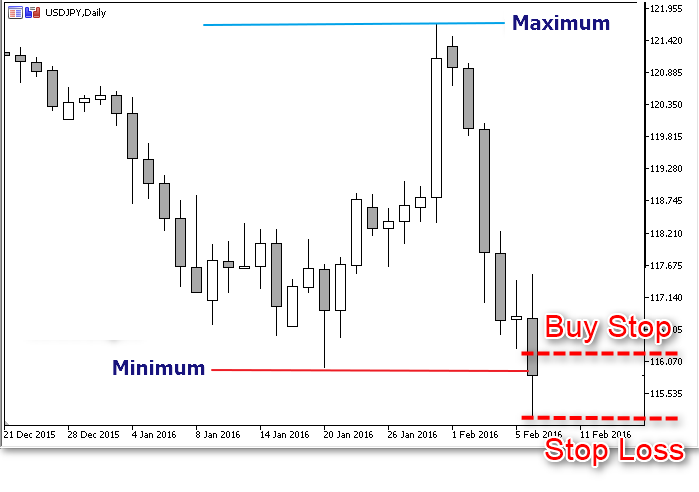The relevant channel limits should be red from the go_Channel structure, and the entry offset specified by the user and then converted to the symbol price is available in the gd_Entry_Offset variable. The calculated level should be validated using the fb_Is_Acceptable_Distance function and the current price value from the go_Tick structure. We will separate these calculations and verifications for BuyStop and SellStop orders:

```double d_Entry_Level = WRONG_VALUE; // The level for placing a pending order
if(e_Signal == ENTRY_BUY) { // For a pending Buy order
// Checking the possibility to place an order:
d_Entry_Level = go_Channel.d_Low + gd_Entry_Offset; // Order placing level
if(!fb_Is_Acceptable_Distance(d_Entry_Level, go_Tick.ask)) {
// The distance from the current price is not enough
if(Log_Level > LOG_LEVEL_ERR)
PrintFormat("BuyStop cannot be placed at the %s level. Bid: %s Ask: %s StopLevel: %s",
DoubleToString(d_Entry_Level, _Digits),
DoubleToString(go_Tick.bid, _Digits),
DoubleToString(go_Tick.ask, _Digits),
DoubleToString(gd_Stop_Level, _Digits)
);

return; // Wait until the current price changes
}
} else {
// Checking the possibility to place an order:
d_Entry_Level = go_Channel.d_High - gd_Entry_Offset; // Order placing level
if(!fb_Is_Acceptable_Distance(d_Entry_Level, go_Tick.bid)) {
// The distance from the current price is not enough
if(Log_Level > LOG_LEVEL_ERR)
PrintFormat("SellStop cannot be placed at the %s level. Bid: %s Ask: %s StopLevel: %s",
DoubleToString(d_Entry_Level, _Digits),
DoubleToString(go_Tick.bid, _Digits),
DoubleToString(go_Tick.ask, _Digits),
DoubleToString(gd_Stop_Level, _Digits)
);

return; // Wait until the current price changes
}
}
```

If the calculated order placing level is successfully verified, we can send the appropriate order to the server using the standard library class:

```// Lot in accordance with the server requirements:
double d_Volume = fd_Normalize_Lot(Trade_Volume);

// Place a pending order:
i_Try = gi_Try_To_Trade;

if(e_Signal == ENTRY_BUY) {
while(i_Try-- > 0) { // Attempts to place BuyStop
if(o_Trade.BuyStop(
d_Volume,
d_Entry_Level,
_Symbol
)) { // Successful attempt
Alert("A pending Buy order has been placed!");
i_Try = -10; // The flag of a successful operation
break;
}
// Failed
Sleep(gi_Connect_Wait); // A pause before the next attempt
}
} else {
while(i_Try-- > 0) { // Attempts to place SellStop
if(o_Trade.SellStop(
d_Volume,
d_Entry_Level,
_Symbol
)) { // Successful attempt
Alert("A pending Sell order has been placed!");
i_Try = -10; // The flag of a successful operation
break;
}
// Failed
Sleep(gi_Connect_Wait); // A pause before the next attempt
}
}

if(i_Try == WRONG_VALUE) // Placing a pending order failed
if(Log_Level > LOG_LEVEL_NONE) Print("Pending order placing error");
```

This is the final step in the Expert Advisor programming. We need to compile it and then we will analyze its performance in the strategy tester.

### Strategy Backtesting

In the book, Connors and Raschke illustrate the strategy using more than 20 year old charts, so the main purpose of testing is to check the strategy performance using more recent years. The source parameters and the daily timeframe specified by the authors were used for testing. 20 years ago, five-digit quotes were not popular, and this testing was performed on five-digit quotes available on the MetaQuotes demo server, so the original indents of 1 and 10 points were transformed into 10 and 100. The original strategy description does not contain trailing parameters, therefore I used the parameters that seemed most appropriate for the daily timeframe.

The graph of Turtle Soup testing results on USDJPY over the last five years: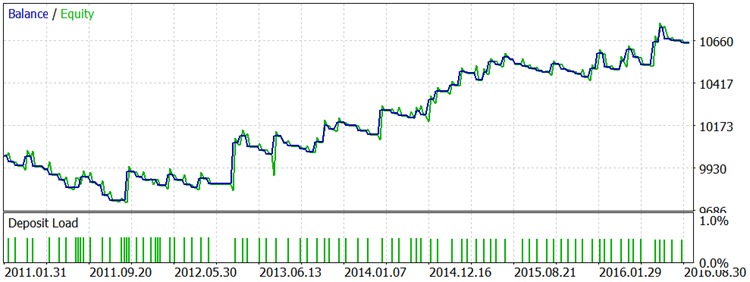The graph of Turtle Soup Plus One testing results with the same parameters on the same history interval of the same instrument: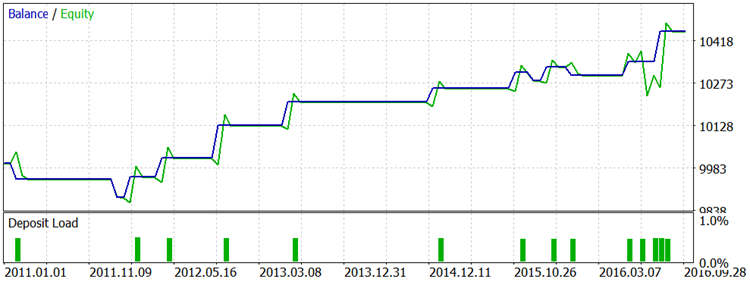The graph of testing results on gold quotes over the last five years: The Turtle Soup strategy: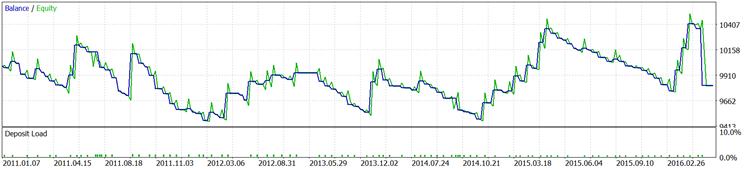Turtle Soup Plus One: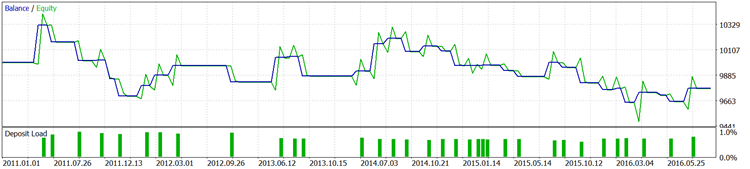The graph of testing results on crude oil quotes over the last four years: The Turtle Soup strategy: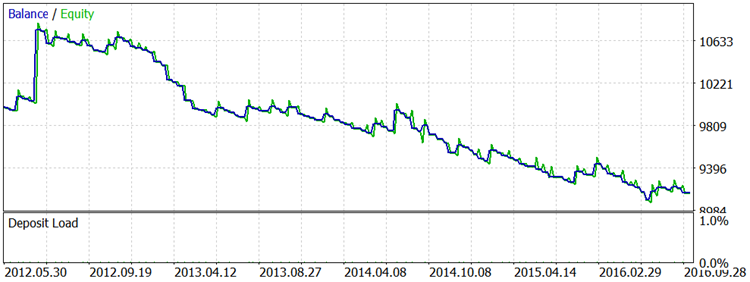Turtle Soup Plus One: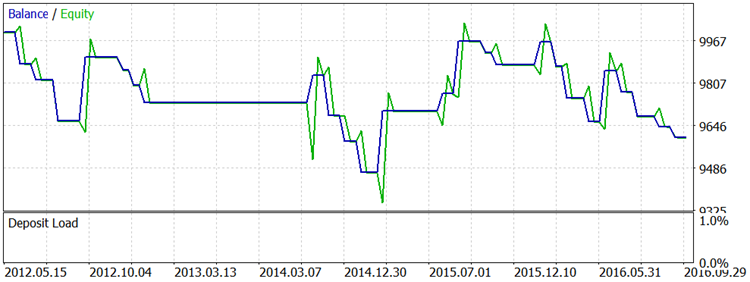The full results of all tests are available in the attached files.

You will do your own conclusions, but I need to give a necessary explanation. Connors and Raschke warn against purely mechanical following of rules of any of the strategies described in the book. They believe it is necessary to analyze how the price approaches the channel borders and how it behaves after testing the limits. Unfortunately, they do not provide any details of that. As for optimization, you can try to adjust parameters for other timeframes, to choose better symbols and parameters.

### Conclusion

We have formalized and programmed the rules of the first pair of trading strategies described in the book Street Smarts: High Probability Short-Term Trading Strategies Trading Strategies — Turtle Soup and Turtle Soup Plus One. The Expert Advisor and the signal library contain all the rules described by Raschke and Connors, but they do not include some of the important details of the authors' trading, which are only briefly mentioned. At least it is necessary to take into accounts gaps and limits of trading sessions. In addition, it seems logical to try to restrict trading by allowing only one entrance per day, or allowing only one profitable entry, allowing to keep a pending order more than up to the beginning of the next day. You can do this if you wish to improve the described Expert Advisor.

Translated from Russian by MetaQuotes Software Corp.
Original article: https://www.mql5.com/ru/articles/2717

Attached files |
MQL5.zip (83.14 KB)
Reports.zip (598.05 KB)

#### Other articles by this author

Last comments | Go to discussion (2)| 10 Feb 2017 at 01:49
Good stuff! Are my eyes playing tricks on me, or are all these different strategies basically unprofitable?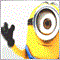| 16 Jul 2017 at 12:54

Good Article , Thank for share..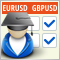LifeHack for Trader: A comparative report of several tests
The article deals with the simultaneous launch of Expert Advisor testing on four different trading instruments. The final comparison of four testing reports is provided in a table similar to how goods are represented in online stores. An additional bonus is that distribution charts will be automatically created for each symbol.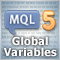MQL5 Programming Basics: Global Variables of the MetaTrader 5 Terminal
Global variables of the terminal provide an indispensable tool for developing sophisticated and reliable Expert Advisors. If you master the global variables, you will no more be able to imagine developing EAs on MQL5 without them.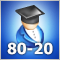80-20 trading strategy
The article describes the development of tools (indicator and Expert Advisor) for analyzing the '80-20' trading strategy. The trading strategy rules are taken from the work "Street Smarts. High Probability Short-Term Trading Strategies" by Linda Raschke and Laurence Connors. We are going to formalize the strategy rules using the MQL5 language and test the strategy-based indicator and EA on the recent market history.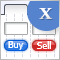Graphical Interfaces X: The Standard Chart Control (build 4)
This time we will consider the Standard chart control. It will allow to create arrays of subcharts with the ability to synchronize horizontal scrolling. In addition, we will continue to optimize the library code to reduce the CPU load.